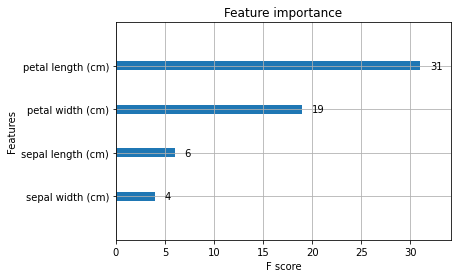# [PYTHON] How to use xgboost: Multi-class classification with iris data

** xgboost ** is a library that handles ** GBDT **, which is a type of decision tree model. We have summarized the steps to install and use. It can be used in various languages, but it describes how to use it in Python.

# What is GBDT

--A type of decision tree model --Gradient boosting tree

Random forest is famous for the same decision tree model, but the following article briefly summarizes the differences. [Machine learning] I tried to summarize the differences between the decision tree models --Qiita

# Features of GBDT

--Easy to get good accuracy --Can handle missing values --Numerical data can be handled

It's easy to use and accurate, so it's popular with Kaggle, a machine learning competition.

#  How to use

I used iris data (iris variety data), which is one of the scikit-learn datasets. The OS is Amazon Linux 2.

## [1-1] Installation

The Amazon Linux 2 I'm using is: The installation procedure for each environment is officially listed. Installation Guide — xgboost 1.1.0-SNAPSHOT documentation

``````pip3 install xgboost
``````

## [1-2] Import

``````import xgboost as xgb
``````

## [1-3] Acquisition of iris data

There are no special steps. Get the iris data and create a DataFrame and Series for pandas.

``````import pandas as pd

iris_data = pd.DataFrame(iris.data, columns=iris.feature_names)
iris_target = pd.Series(iris.target)
``````

## [1-4] Acquisition of training data and test data

Again, there are no special steps, and scikit-learn's `train_test_split` splits the data for training and testing.

``````from sklearn.model_selection import train_test_split

train_x, test_x, train_y, test_y = train_test_split(iris_data, iris_target, test_size=0.2, shuffle=True)
``````

## [1-5] Convert to type for xgboost

xgboost uses `DMatrix`.

``````dtrain = xgb.DMatrix(train_x, label=train_y)
``````

`DMatrix` can be created from numpy's` ndarray` or pandas'`DataFrame`, so you won't have any trouble handling the data.

The types of data that can be handled are officially detailed. Python Package Introduction — xgboost 1.1.0-SNAPSHOT documentation

## [1-6] Parameter setting

Set various parameters.

``````param = {'max_depth': 2, 'eta': 1, 'objective': 'multi:softmax', 'num_class': 3}
``````

The meaning of each parameter is as follows.

Parameter name meaning
max_depth Maximum depth of the tree
eta Learning rate
objective Learning purpose
num_class Number of classes

Specify the learning purpose (regression, classification, etc.) in'objejective'. Since this time it is a multi-class classification,'multi: softmax' is specified.

Details are officially detailed. XGBoost Parameters — xgboost 1.1.0-SNAPSHOT documentation

## [1-7] Learning

`num_round` is the number of learnings.

``````num_round = 10
bst = xgb.train(param, dtrain, num_round)
``````

## [1-8] Forecast

``````dtest = xgb.DMatrix(test_x)
pred = bst.predict(dtest)
``````

## [1-9] Confirmation of accuracy

Check the accuracy rate with ʻaccuracy_score` in scikit-learn.

``````from sklearn.metrics import accuracy_score

score = accuracy_score(test_y, pred)
print('score:{0:.4f}'.format(score))
# 0.9667
``````

## [1-10] Visualization of importance

Visualize which features contributed to the prediction results.

``````xgb.plot_importance(bst)
``````#  Validation and early stopping during learning

You can easily perform validation during learning using verification data and early stopping (discontinuation of learning).

## [2-1] Data division

A part of the training data is used as verification data.

``````train_x, valid_x, train_y, valid_y = train_test_split(train_x, train_y, test_size=0.2, shuffle=True)
``````

## [2-2] Creating DMatrix

``````dtrain = xgb.DMatrix(train_x, label=train_y)
dvalid = xgb.DMatrix(valid_x, label=valid_y)
``````

Add'eval_metric' to the parameter for validation. For'eval_metric', specify the metric.

``````param = {'max_depth': 2, 'eta': 0.5, 'objective': 'multi:softmax', 'num_class': 3, 'eval_metric': 'mlogloss'}
``````

## [2-4] Learning

Specify the data to be monitored by validation in evallist. Specify'eval'as the name of the verification data and'train' as the name of the training data.

I'm adding ʻearly_stopping_rounds` as an argument to xgb.train. ʻEarly_stopping_rounds = 5` means that learning will be stopped if the evaluation index does not improve 5 times in a row.

``````evallist = [(dvalid, 'eval'), (dtrain, 'train')]
num_round = 10000
bst = xgb.train(param, dtrain, num_round, evallist, early_stopping_rounds=5)
# 	eval-mlogloss:0.61103	train-mlogloss:0.60698
# Multiple eval metrics have been passed: 'train-mlogloss' will be used for early stopping.
#
# Will train until train-mlogloss hasn't improved in 5 rounds.
# 	eval-mlogloss:0.36291	train-mlogloss:0.35779
# 	eval-mlogloss:0.22432	train-mlogloss:0.23488
#
#~ ~ ~ Omitted on the way ~ ~ ~
#
# Stopping. Best iteration:
# 	eval-mlogloss:0.00827	train-mlogloss:0.01863
``````

## [2-5] Confirmation of verification results

``````print('Best Score:{0:.4f}, Iteratin:{1:d}, Ntree_Limit:{2:d}'.format(
bst.best_score, bst.best_iteration, bst.best_ntree_limit))
# Best Score:0.0186, Iteratin:1153, Ntree_Limit:1154
``````

## [2-6] Forecast

Make predictions using the model with the best verification results.

``````dtest = xgb.DMatrix(test_x)
pred = ypred = bst.predict(dtest, ntree_limit=bst.best_ntree_limit)
``````

# At the end

Since I can use pandas' DataFrame and Series, I felt that the threshold was low for those who have been doing machine learning so far.

I tried multi-class classification this time, but it can also be used for binary classification and regression, so it can be used in various situations.